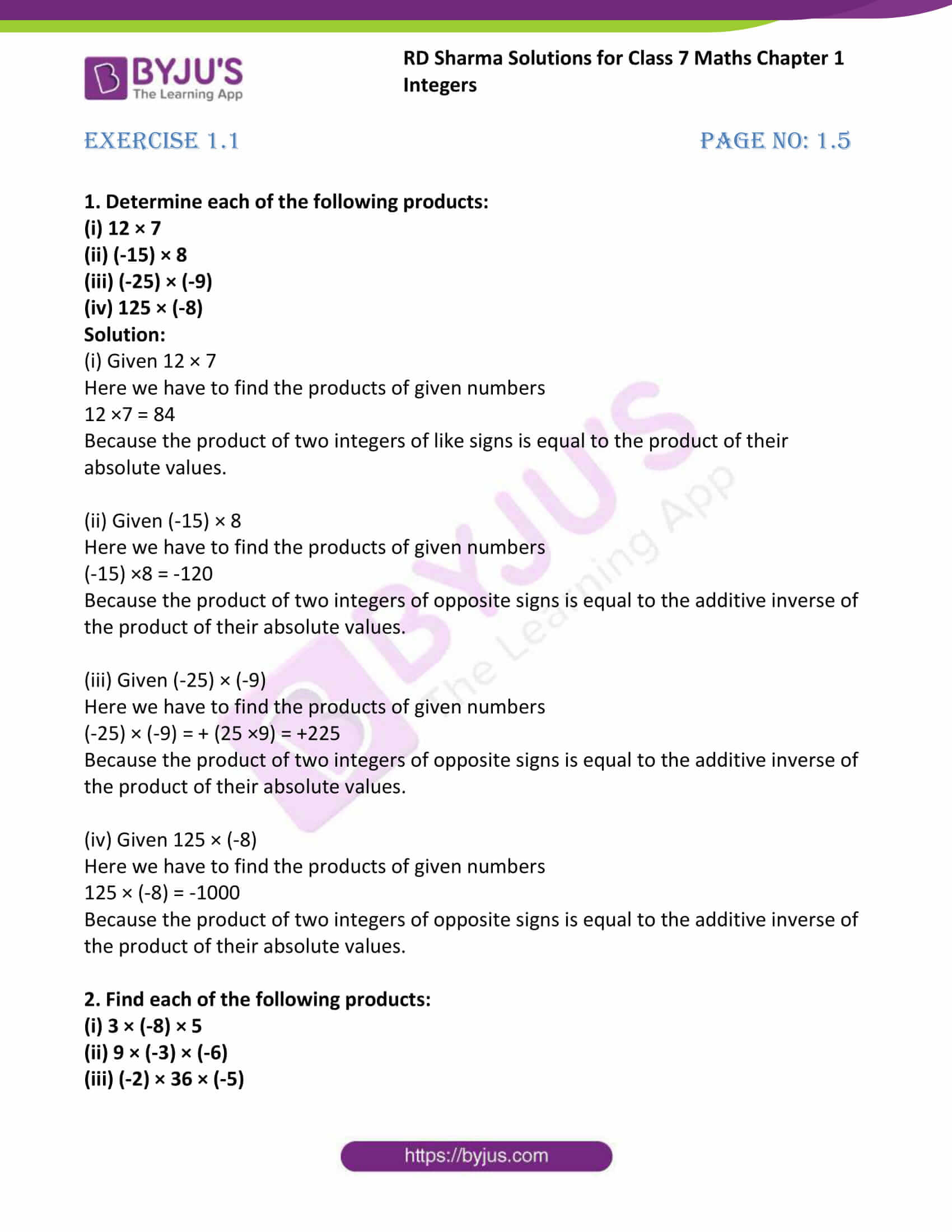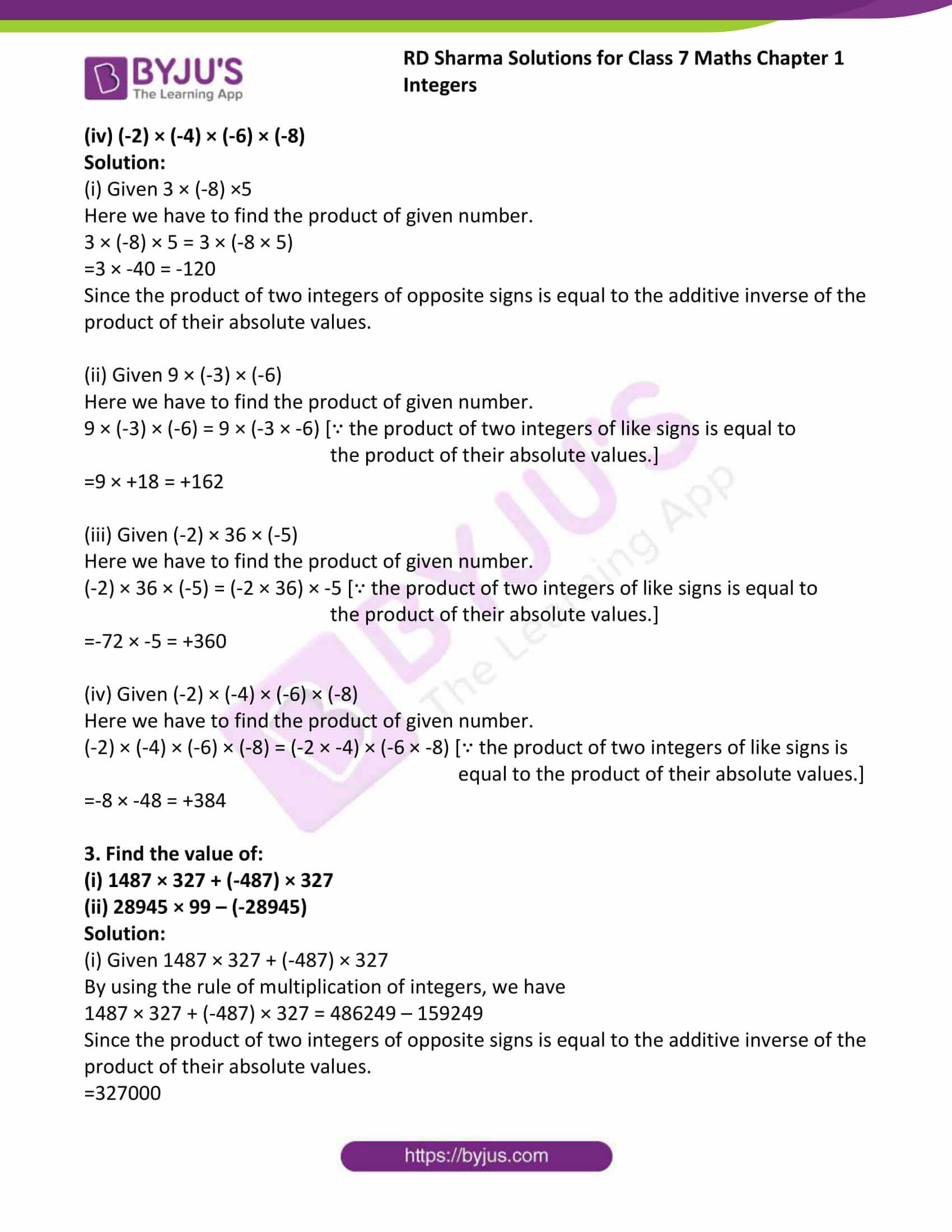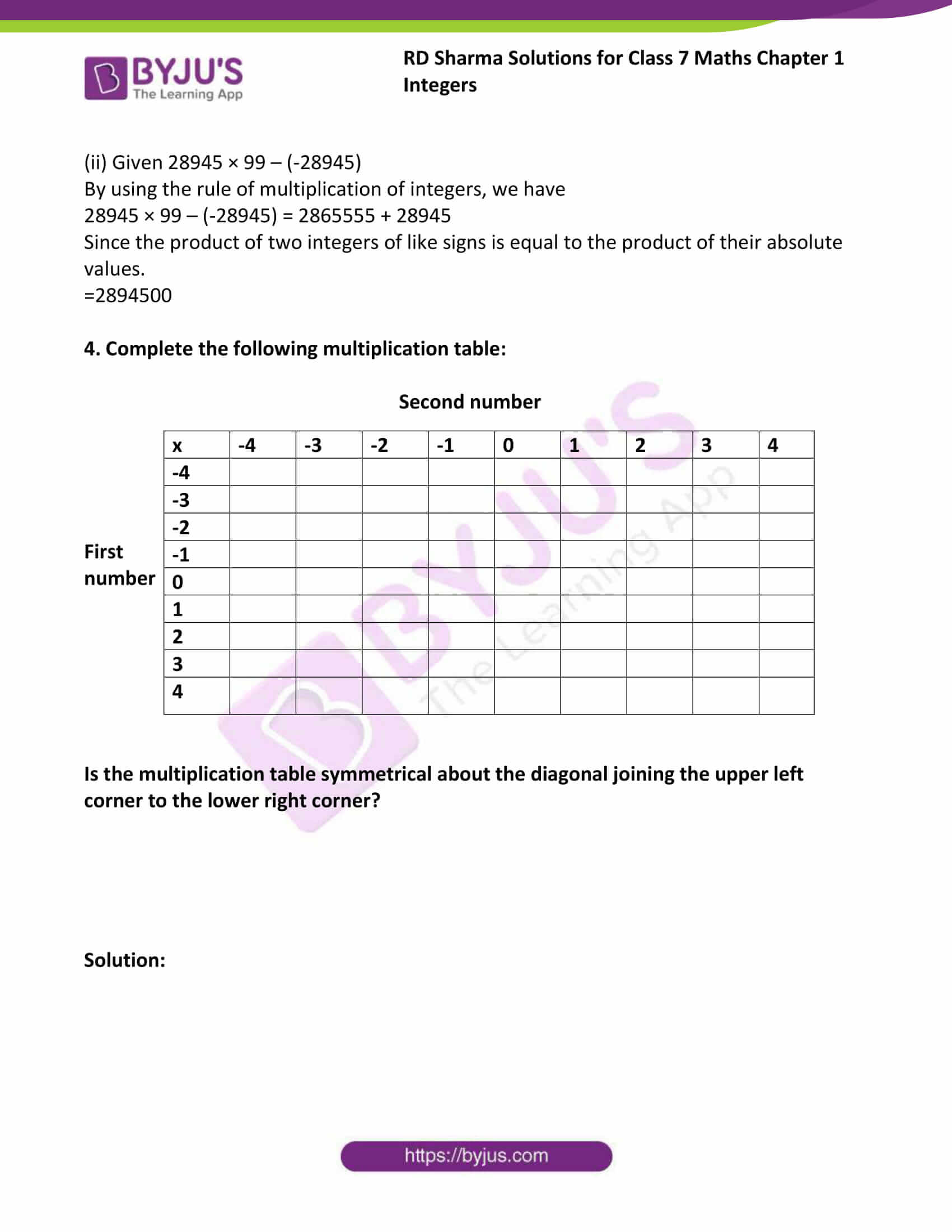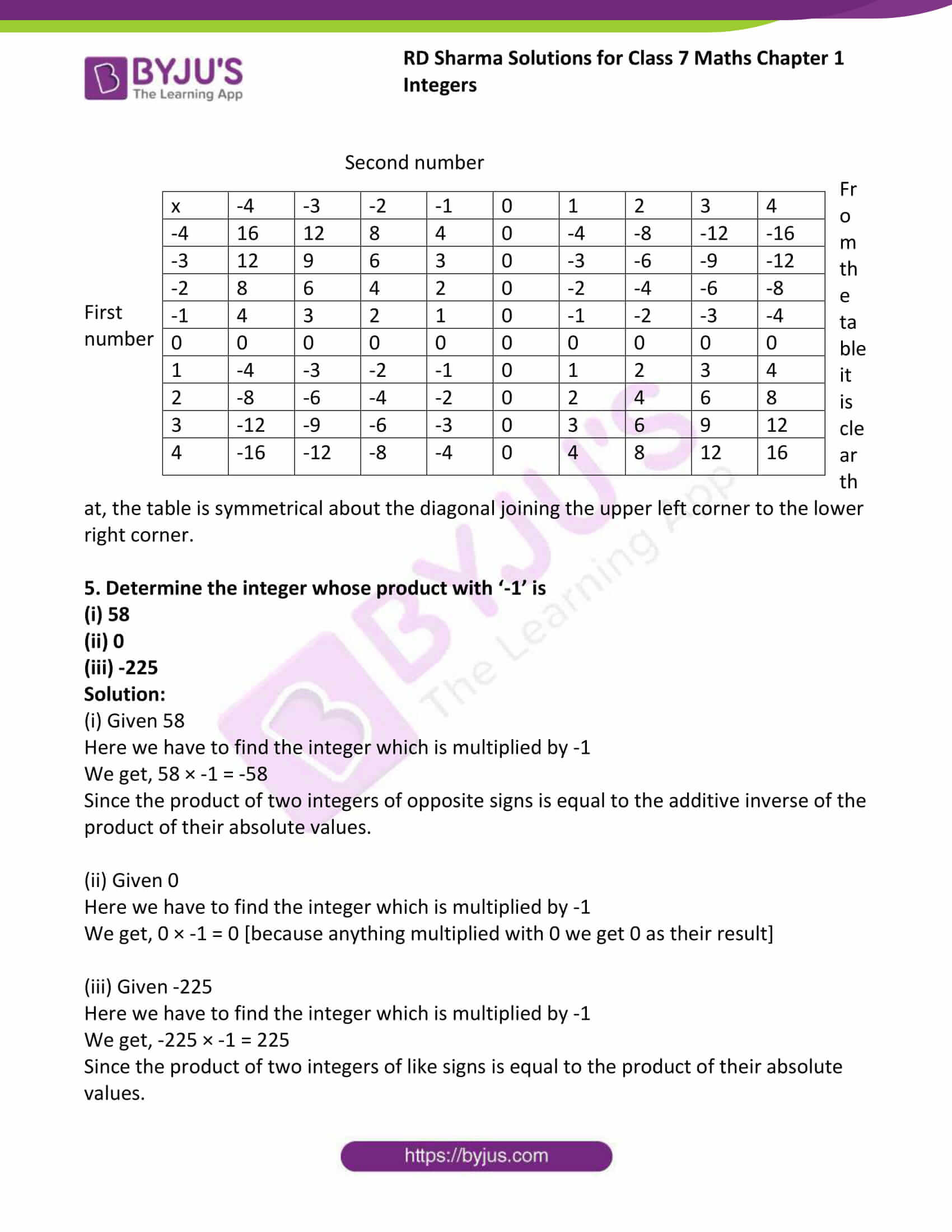# RD Sharma Solutions For Class 7 Maths Chapter 1 Integers Exercise 1.1

The PDF of RD Sharma Solutions for Exercise 1.1 of Class 7 Maths Chapter 1 Integers are provided here. The questions present in this have been solved by BYJU’S experts in Maths, and this will help students solve the problems without any difficulties. Exercise 1.1 contains ten questions with many sub-questions which deals with the multiplication of integers and their properties. Students can obtain worthy results in Class 7 exams by using the RD Sharma Solutions. By practising the RD Sharma Solutions for Class 7, students will be able to grasp the concepts correctly.

## Download the PDF of RD Sharma Solutions For Class 7 Maths Chapter 1 – Integers Exercise 1.1### Access answers to Maths RD Sharma Solutions For Class 7 Chapter 1 – Integers Exercise 1.1

Exercise 1.1 Page No: 1.5

1. Determine each of the following products:

(i) 12 × 7

(ii) (-15) × 8

(iii) (-25) × (-9)

(iv) 125 × (-8)

Solution:

(i) Given 12 × 7

Here we have to find the products of given numbers

12 ×7 = 84

Because the product of two integers of like signs is equal to the product of their absolute values.

(ii) Given (-15) × 8

Here we have to find the products of given numbers

(-15) ×8 = -120

Because the product of two integers of opposite signs is equal to the additive inverse of the product of their absolute values.

(iii) Given (-25) × (-9)

Here we have to find the products of given numbers

(-25) × (-9) = + (25 ×9) = +225

Because the product of two integers of opposite signs is equal to the additive inverse of the product of their absolute values.

(iv) Given 125 × (-8)

Here we have to find the products of given numbers

125 × (-8) = -1000

Because the product of two integers of opposite signs is equal to the additive inverse of the product of their absolute values.

2. Find each of the following products:

(i) 3 × (-8) × 5

(ii) 9 × (-3) × (-6)

(iii) (-2) × 36 × (-5)

(iv) (-2) × (-4) × (-6) × (-8)

Solution:

(i) Given 3 × (-8) ×5

Here we have to find the product of given number.

3 × (-8) × 5 = 3 × (-8 × 5)

=3 × -40 = -120

Since the product of two integers of opposite signs is equal to the additive inverse of the product of their absolute values.

(ii) Given 9 × (-3) × (-6)

Here we have to find the product of given number.

9 × (-3) × (-6) = 9 × (-3 × -6) [∵ the product of two integers of like signs is equal to

the product of their absolute values.]

=9 × +18 = +162

(iii) Given (-2) × 36 × (-5)

Here we have to find the product of given number.

(-2) × 36 × (-5) = (-2 × 36) × -5 [∵ the product of two integers of like signs is equal to

the product of their absolute values.]

=-72 × -5 = +360

(iv) Given (-2) × (-4) × (-6) × (-8)

Here we have to find the product of given number.

(-2) × (-4) × (-6) × (-8) = (-2 × -4) × (-6 × -8) [∵ the product of two integers of like signs is

equal to the product of their absolute values.]

=-8 × -48 = +384

3. Find the value of:

(i) 1487 × 327 + (-487) × 327

(ii) 28945 × 99 – (-28945)

Solution:

(i) Given 1487 × 327 + (-487) × 327

By using the rule of multiplication of integers, we have

1487 × 327 + (-487) × 327 = 486249 – 159249

Since the product of two integers of opposite signs is equal to the additive inverse of the product of their absolute values.

=327000

(ii) Given 28945 × 99 – (-28945)

By using the rule of multiplication of integers, we have

28945 × 99 – (-28945) = 2865555 + 28945

Since the product of two integers of like signs is equal to the product of their absolute values.

=2894500

4. Complete the following multiplication table:

Second number

 First number x -4 -3 -2 -1 0 1 2 3 4 -4 -3 -2 -1 0 1 2 3 4

Is the multiplication table symmetrical about the diagonal joining the upper left corner to the lower right corner?

Solution:

Second number

 First number x -4 -3 -2 -1 0 1 2 3 4 -4 16 12 8 4 0 -4 -8 -12 -16 -3 12 9 6 3 0 -3 -6 -9 -12 -2 8 6 4 2 0 -2 -4 -6 -8 -1 4 3 2 1 0 -1 -2 -3 -4 0 0 0 0 0 0 0 0 0 0 1 -4 -3 -2 -1 0 1 2 3 4 2 -8 -6 -4 -2 0 2 4 6 8 3 -12 -9 -6 -3 0 3 6 9 12 4 -16 -12 -8 -4 0 4 8 12 16

From the table it is clear that, the table is symmetrical about the diagonal joining the upper left corner to the lower right corner.

5. Determine the integer whose product with ‘-1’ is

(i) 58

(ii) 0

(iii) -225

Solution:

(i) Given 58

Here we have to find the integer which is multiplied by -1

We get, 58 × -1 = -58

Since the product of two integers of opposite signs is equal to the additive inverse of the product of their absolute values.

(ii) Given 0

Here we have to find the integer which is multiplied by -1

We get, 0 × -1 = 0 [because anything multiplied with 0 we get 0 as their result]

(iii) Given -225

Here we have to find the integer which is multiplied by -1

We get, -225 × -1 = 225

Since the product of two integers of like signs is equal to the product of their absolute values.

## RD Sharma Solutions for Class 7 Maths Chapter – 1 Integers Exercise 1.1

Exercise 1.1 of RD Sharma Solutions for Chapter 1, Integers deals with the basic concepts related to Integers. Some of the topics focused prior to Exercise 1.1 are listed below.

• Multiplication of integers
• Properties of multiplication

Students are suggested to try solving the questions from RD Sharma book of Class 7 and then refer to these solutions to know the best way of approaching the different questions.